# Constructing a Triangle When the Length of Its three Sides Are Known (SSS Criterion):

We would construct triangles when all its sides are known. We draw first a rough sketch to give an idea of where the sides are and then begin by drawing any one of the three lines.

1) Construct a triangle ABC, given that AB = 5 cm, BC = 6 cm and AC = 7 cm.

Step 1: First, we draw a rough sketch with a given measure.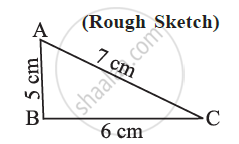Step 2: Draw a line segment BC of length 6 cm.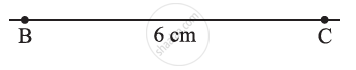Step 3: From B, point A is at a distance of 5 cm. So, with B as center, draw an arc of radius 5 cm.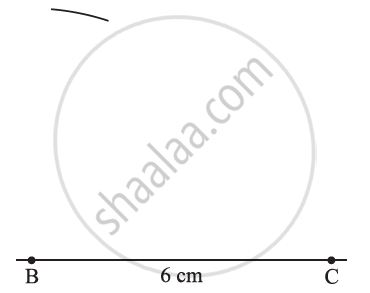Step 4: From C, point A is at a distance of 7 cm. So, with C as the centre, draw an arc of  radius 7 cm.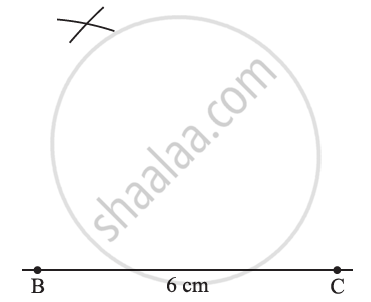Step 5: A has to be on both the arcs drawn. So, it is the point of intersection of arcs. Mark the point of intersection of arcs as A. Join AB and AC. ∆ABC is now ready.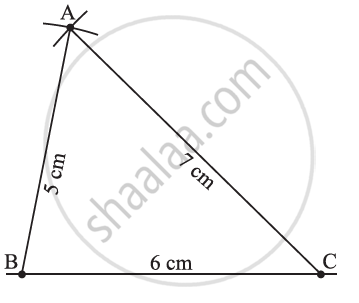We observe that ∆DEF exactly coincides with ∆ABC.

1) Draw ΔXYZ such that l(XY) = 6 cm, l(YZ) = 4 cm, l(XZ) = 5 cm.

Let us draw a rough figure quickly and show the given information in it as accurately as possible. For example, side XY is the longest, so, in the rough figure, too, XY should be the longest side.

## Steps:

1. According to the rough figure, segment XY of length 6 cm is drawn as the base.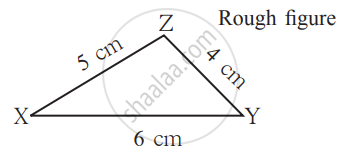2. As l(XZ) is 5 cm, draw an arc on one side of seg XY with the compass opened to 5 cm and with its point at X.

3. Next, with the point at Y and the compass opened to 4 cm, draw an arc to cut the first arc at Z. Draw segs XY and YZ.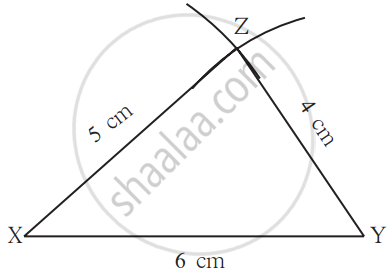4. A similar construction can be drawn on the other side of the base as shown below.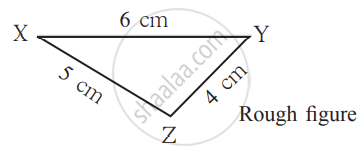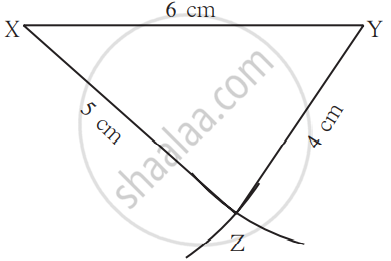Thus, if three sides of one triangle are equal to the corresponding three sides of another triangle, then the two triangles are congruent. This is SSS congruency rule.
If you would like to contribute notes or other learning material, please submit them using the button below.

### Shaalaa.com

Constructing a Triangle When the Lengh of Its Sides Are Known (SSS Criterion) [00:01:16]
S
0%